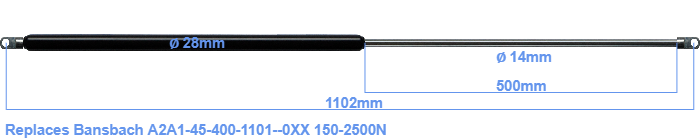# Replacement for Bansbach A2A1-45-400-1101–0XX 150-2500N

\$77.50

Replacement gas spring for the Bansbach A2A1-45-400-1101--0XX 150-2500 Newton. The eye has a thickness of 10mm and a hole diameter of 8.1mm. Brand: HAHN, a Stabilus company.

Please note:
Mounting part rod : The thickness of the eye of this replacement is 10mm instead of 18mm.
 Force Choose an option150 Newton200 Newton250 Newton300 Newton350 Newton400 Newton450 Newton500 Newton550 Newton600 Newton650 Newton700 Newton750 Newton800 Newton850 Newton900 Newton950 Newton1000 Newton1050 Newton1100 Newton1150 Newton1200 Newton1250 Newton1300 Newton1350 Newton1400 Newton1450 Newton1500 Newton1550 Newton1600 Newton1650 Newton1700 Newton1750 Newton1800 Newton1850 Newton1900 Newton1950 Newton2000 Newton2050 Newton2100 Newton2150 Newton2200 Newton2250 Newton2300 Newton2350 Newton2400 Newton2450 Newton2500 NewtonClearThe bore of the house of this replacement gas spring is 28 mm. The diameter of the rod totals 14 mm. The stroke of the gas spring is 500 millimeter. In total, the length is 1102 millimeter. Note: this is the total length between the rotation points of the two mounting parts. The length from thread to thread (wihout mounting parts) is 1048 mm. The force of this replacement is 150-2500 Newton. Attention: this is not an official Bansbach gas spring, but a replacement gas spring. This gas spring was produced by Stabilus subsidiary HAHN. Nevertheless, this gas spring can be used as replacement gas spring, because the dimensions and forces are almost entirely equal.